# prWarp: Papionin example

#### 2020-10-22

This document corresponds to the worked example of the paper Detecting phylogenetic signal and adaptation in papionin cranial shape by decomposing variation at different spatial scales (Grunstra et al., in press). The dataset is a set of 70 2D landmarks on the midsagittal plane of the skull in 67 primates from 18 species (16 papionin taxa, 2 outgroups). The example below describes how to decompose shape variation into total, outline, and residual shape (approach 1) or into large-scale and small-scale shape (approach 2). For further details, please read the associated paper.

# Data preparation

Install and load packages in R.

``````library("prWarp")
library("geomorph")``````

Load the dataset `papionin`. This dataset comprises the coordinates of 70 two-dimensional landmarks for the 67 specimens as a 3D array.

``````data("papionin")  # load dataset
species <- papionin\$species  # species
papionin_ar <- papionin\$coords  # landmark coordinates
k <- dim(papionin_ar)  # number of landmarks
n_spec <- dim(papionin_ar)  # number of specimens
n_species <- length(levels(species))  # number of species``````

# Approach 1: Total, outline, and residual shape variation

In this approach the total shape variation is decomposed into the variation of outline shape and residual shape. The outline shape corresponds to a subset of the full landmark set, in which all landmarks that demarcate the boarders between bones (i.e., landmarks on sutures and synchondroses) are free to slide. The residual shape corresponds to the slid landmark coordinates of the full dataset after warping them to the mean outline shape using TPS interpolation.

## Total shape

For the full landmark set (70 landmarks), define semilandmarks and curves for landmark sliding as well as links between landmarks for visualization.

``````# Semilandmarks
all_semi_lm <- papionin\$semi_lm
# Curves for the sliding process
all_curves <- papionin\$curves
# Links between landmarks for visualization

Visualize fixed (red) vs. sliding (black) landmarks for one specimen before sliding.

``````plot(papionin_ar[,,1], pch = 16, col = "black", cex = 0.7, asp = 1)
points(papionin_ar[-all_semi_lm,,1], pch = 16, col = "red")
text(papionin_ar[-all_semi_lm,,1], label = c(1:k)[-all_semi_lm], pos = 1, col = "red", cex = 0.8)
text(papionin_ar[all_semi_lm,,1], label = all_semi_lm, pos = 1, col = "black", cex = 0.6)``````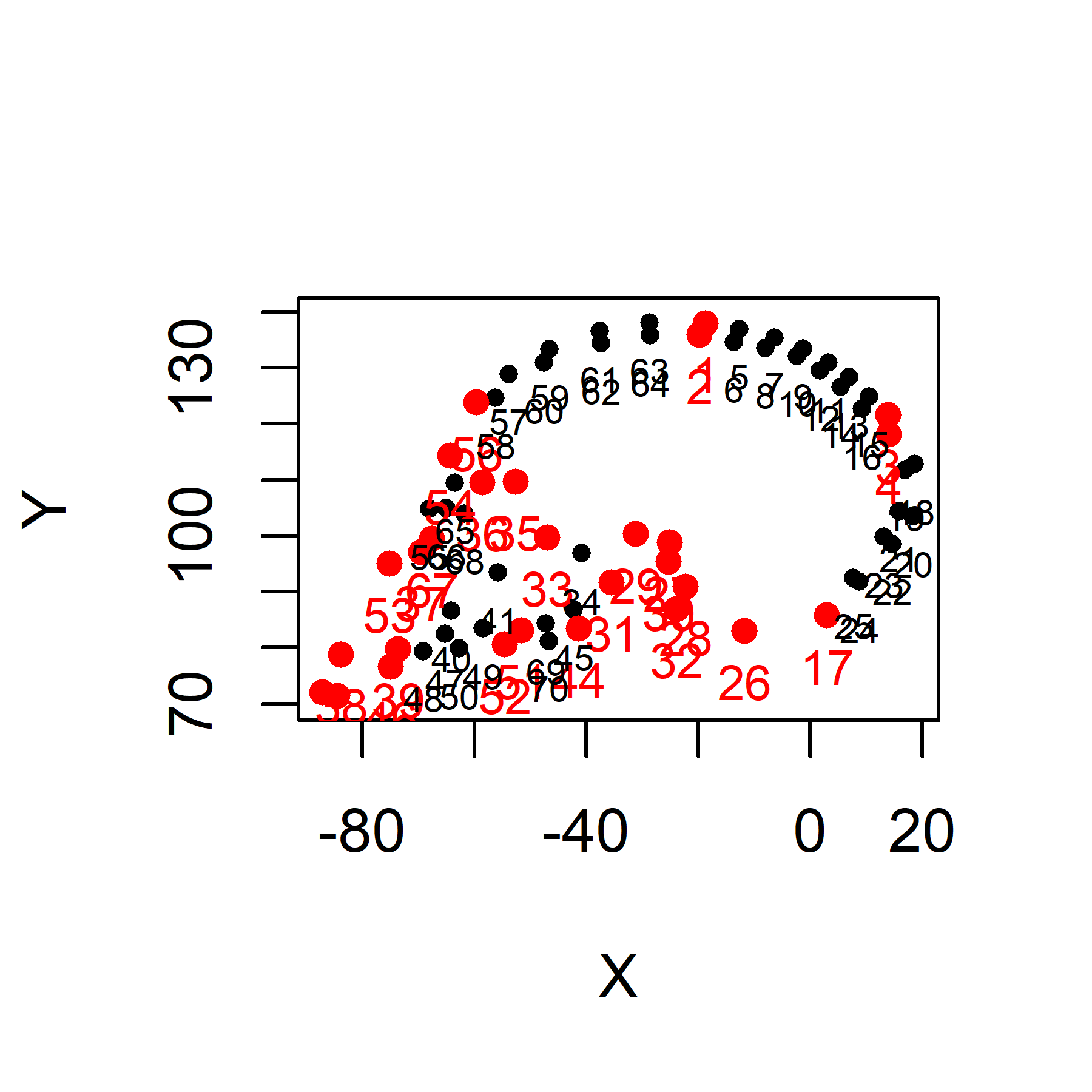Slide the 70 landmarks by minimizing bending energy. Note that the `procSym` function of the package `Morpho` gives as output both slid but not Procrustes aligned outline landmarks (i.e., slid landmarks in the original coordinate system) and slid and Procrustes aligned outline landmarks (i.e., slid landmarks superimposed for all specimens in a new coordinate system).

``papionin_gpa <- Morpho::procSym(papionin_ar, SMvector = all_semi_lm, outlines = all_curves, pcAlign = FALSE)  # sliding + GPA``
``````## reflection involved
## Iteration 1 ..
## squared distance between means: 3.83893855864639
##  -------------------------------------------
## Iteration 2 ..
## squared distance between means: 7.72205177923358e-05
##  -------------------------------------------
## Iteration 3 ..
## squared distance between means: 3.33008920865188e-06
##  -------------------------------------------``````
``````papionin_ar_slid <- papionin_gpa\$dataslide  # slid landmarks in the original space
total_shape <- papionin_gpa\$rotated  # Procrustes coordinates
total_shape_average <- papionin_gpa\$mshape  # average shape``````

Visualize fixed (red) vs. sliding (black) landmarks for one specimen after sliding, for comparisons to the position of the semilandmarks before sliding.

``````plot(papionin_ar_slid[,,1], pch = 16, col = "black", cex = 0.7, asp = 1)
points(papionin_ar_slid[-all_semi_lm,,1], pch = 16, col = "red")
text(papionin_ar_slid[-all_semi_lm,,1], label = c(1:k)[-all_semi_lm], pos = 1, col = "red", cex = 0.8)
text(papionin_ar_slid[all_semi_lm,,1], label = all_semi_lm, pos = 1, col = "black", cex = 0.6)``````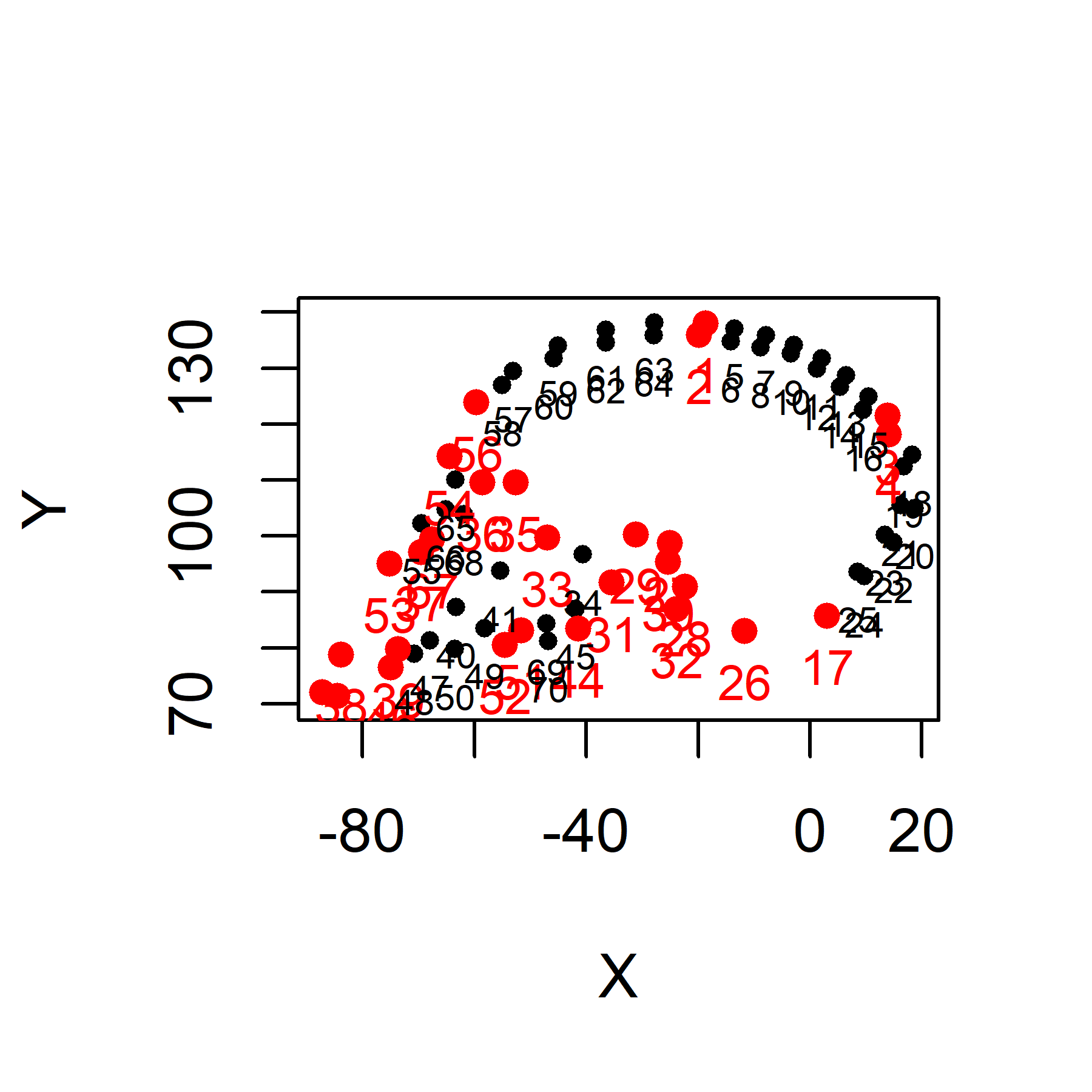For the analyses of total shape variation use slid landmarks after superimposition (generalized Procrustes analysis, GPA).

Total shape variation for all specimens.

``````plotAllSpecimens(total_shape, mean = FALSE, plot.param = list(pt.cex = 0.5))
for (i in 1:n_spec) {
}``````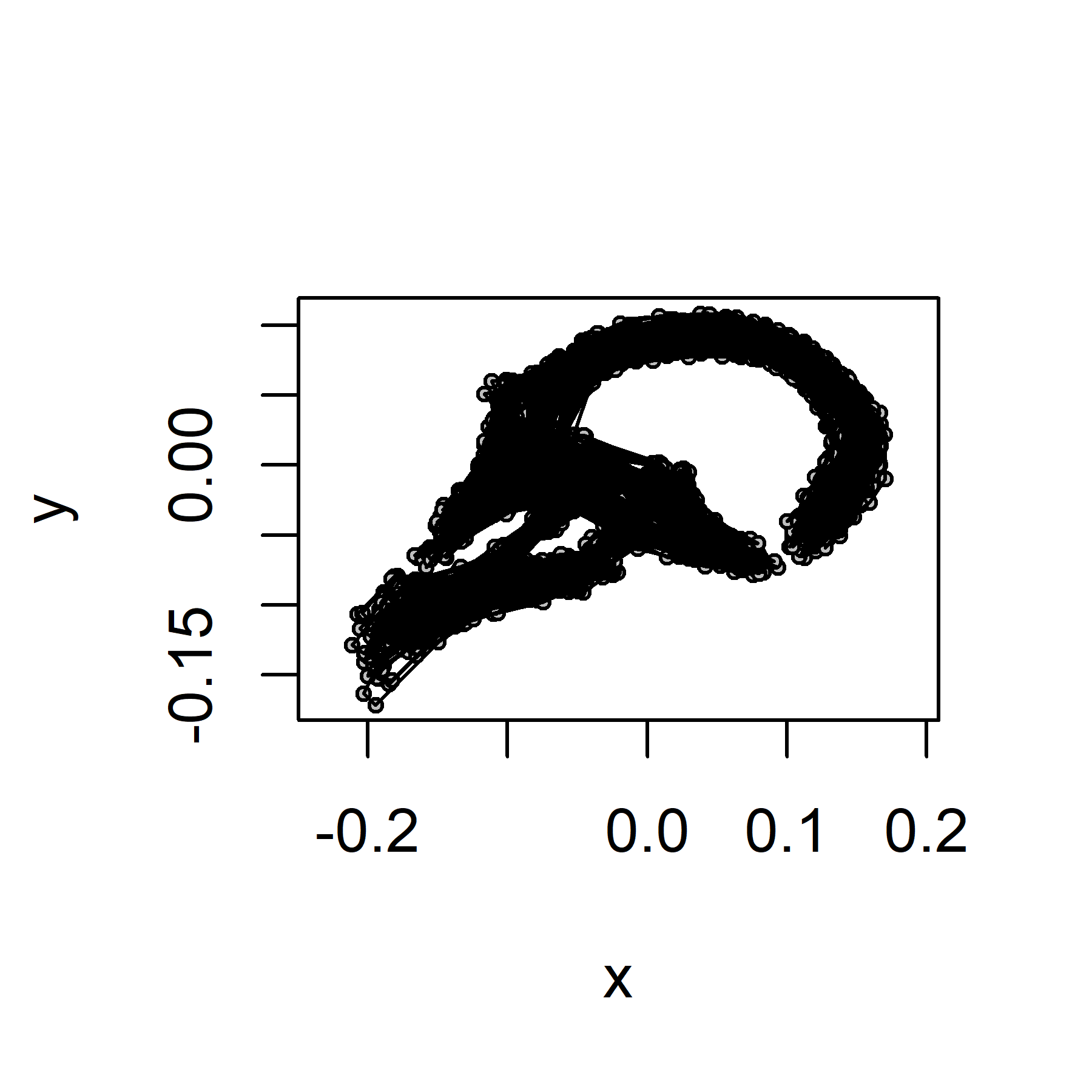Total shape variation between species.

``````# Computation of the average total shape for each species
total_shape_between <- array(NA, dim = c(k, 2, n_species))
for (i in 1:n_species) {
spi <- which(species == levels(species)[i])
total_shape_between[,,i] <- mshape(total_shape[, , spi])
}
# Plot total shape between species
plotAllSpecimens(total_shape_between, mean = FALSE, plot.param = list(pt.cex = 0.5))
for (i in 1:n_species) {
}``````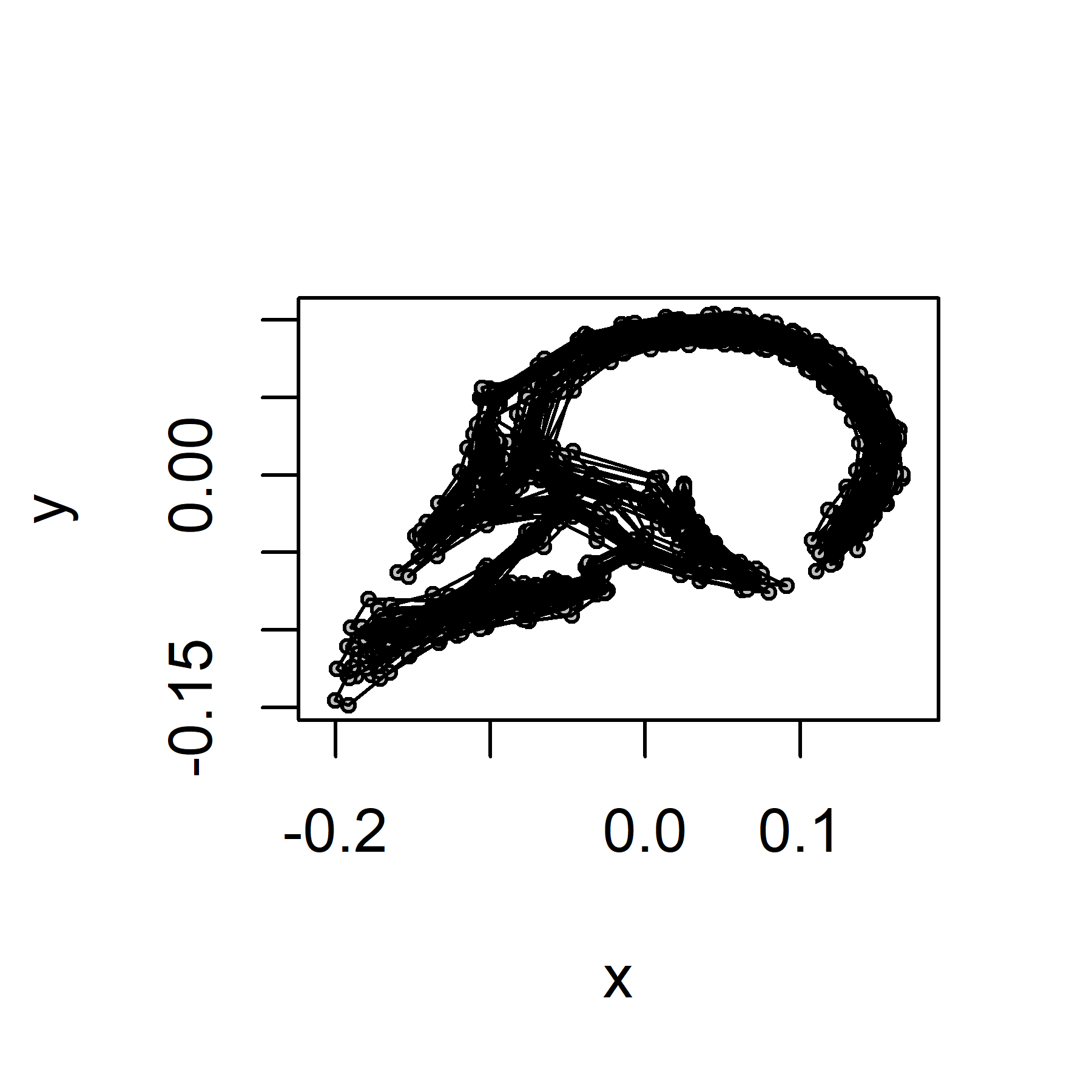Total shape variation within species.

``````# Computation of the pooled individual within-species total shape
total_shape_within <- array(NA, dim = c(k, 2, n_spec))
for (i in 1:n_species) {
spi <- which(species == levels(species)[i])
for (j in 1:length(spi)) {
total_shape_within[,,spi[j]] <- total_shape[, , spi[j]] - total_shape_between[,,i] + mshape(total_shape_between)
}
}
# Plot the pooled individual within-species total shape
plotAllSpecimens(total_shape_within, mean = FALSE, plot.param = list(pt.cex = 0.5))
for (i in 1:n_spec) {
}``````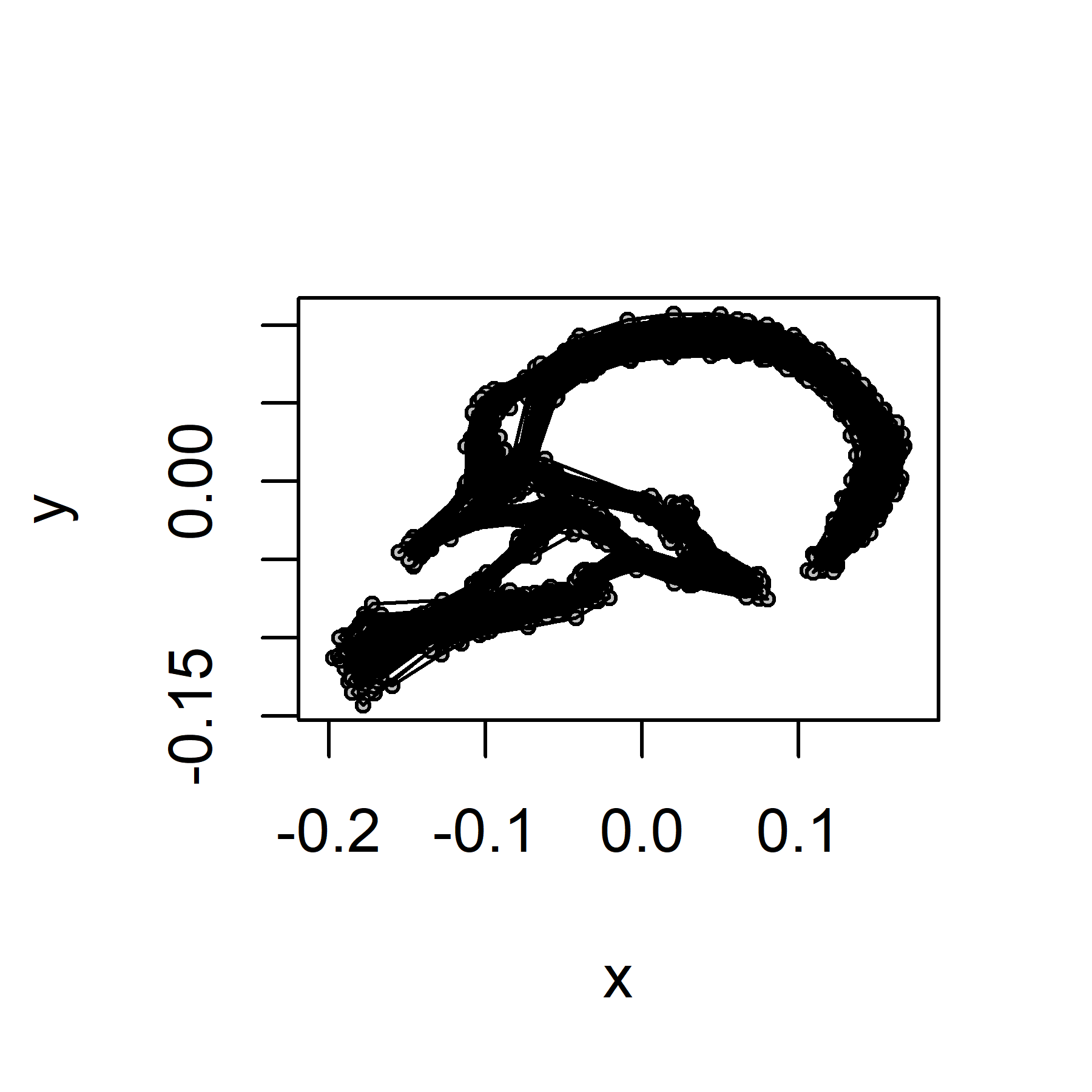## Outline shape

The 'outline' shape corresponds to a subset of 54 landmarks, of which most are defined as semilandmarks and hence free to slide.

``````outline_lm <- papionin\$outline\$subset  # subset
k_out <- length(outline_lm)  # number of landmarks in the subset
outline_ar <- papionin_ar[outline_lm, , ]  # select the subset of landmark coordinates``````

Visualize the landmarks selected to define the subset (in blue).

``````plot(papionin_ar[outline_lm,,1], pch = 16, col = "blue", asp = 1)
points(papionin_ar[-outline_lm,,1], pch = 4, col = "black", cex = 0.7)
text(papionin_ar[outline_lm,,1], label = outline_lm, pos = 1, col = "blue", cex = 0.8)
text(papionin_ar[-outline_lm,,1], label = c(1:k_out)[-outline_lm], pos = 1, col = "black", cex = 0.6)``````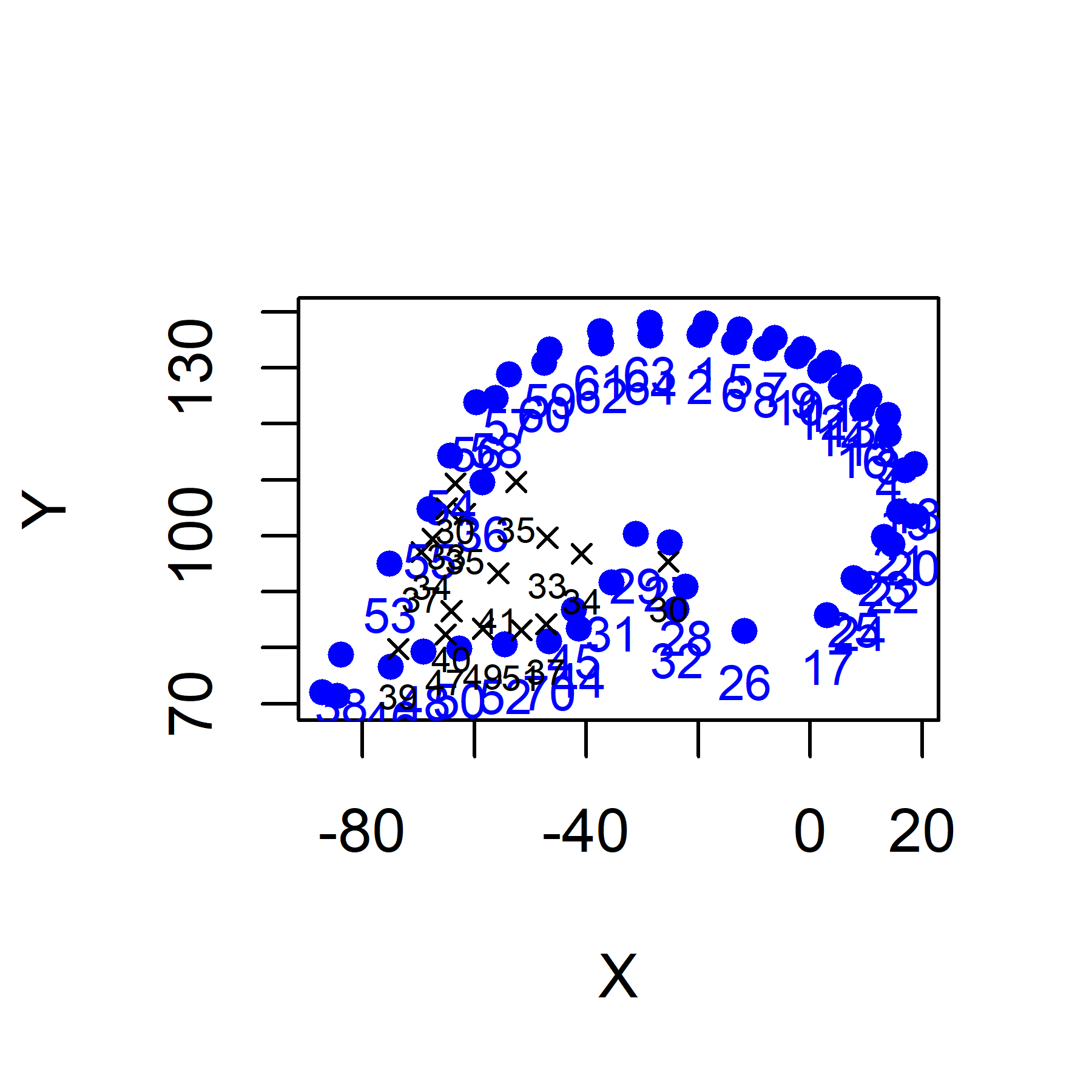For the outline landmark set (54 landmarks), define semilandmarks and curves for landmark sliding as well as links between landmarks for visualization. Note that the landmark numbers refer to the order in the 54-landmark set and not in the initial 70-landmarks set.

``````# Semilandmarks
outline_semi_lm <- papionin\$outline\$semi_lm
# Curves
outline_curves <- papionin\$outline\$curves
# Links between landmarks (for visualization)

Visualize fixed (red) vs. sliding (black) landmarks for the outline of one specimen before sliding.

``````plot(outline_ar[,,1], pch = 16, col = "black", cex = 0.7, asp = 1)
points(outline_ar[-outline_semi_lm,,1], pch = 16, col = "red")
text(outline_ar[outline_semi_lm,,1], label = outline_semi_lm, pos = 1, col = "black", cex = 0.6)
text(outline_ar[-outline_semi_lm,,1], label = c(1:k_out)[-outline_semi_lm], pos = 1, col = "red", cex = 0.8)``````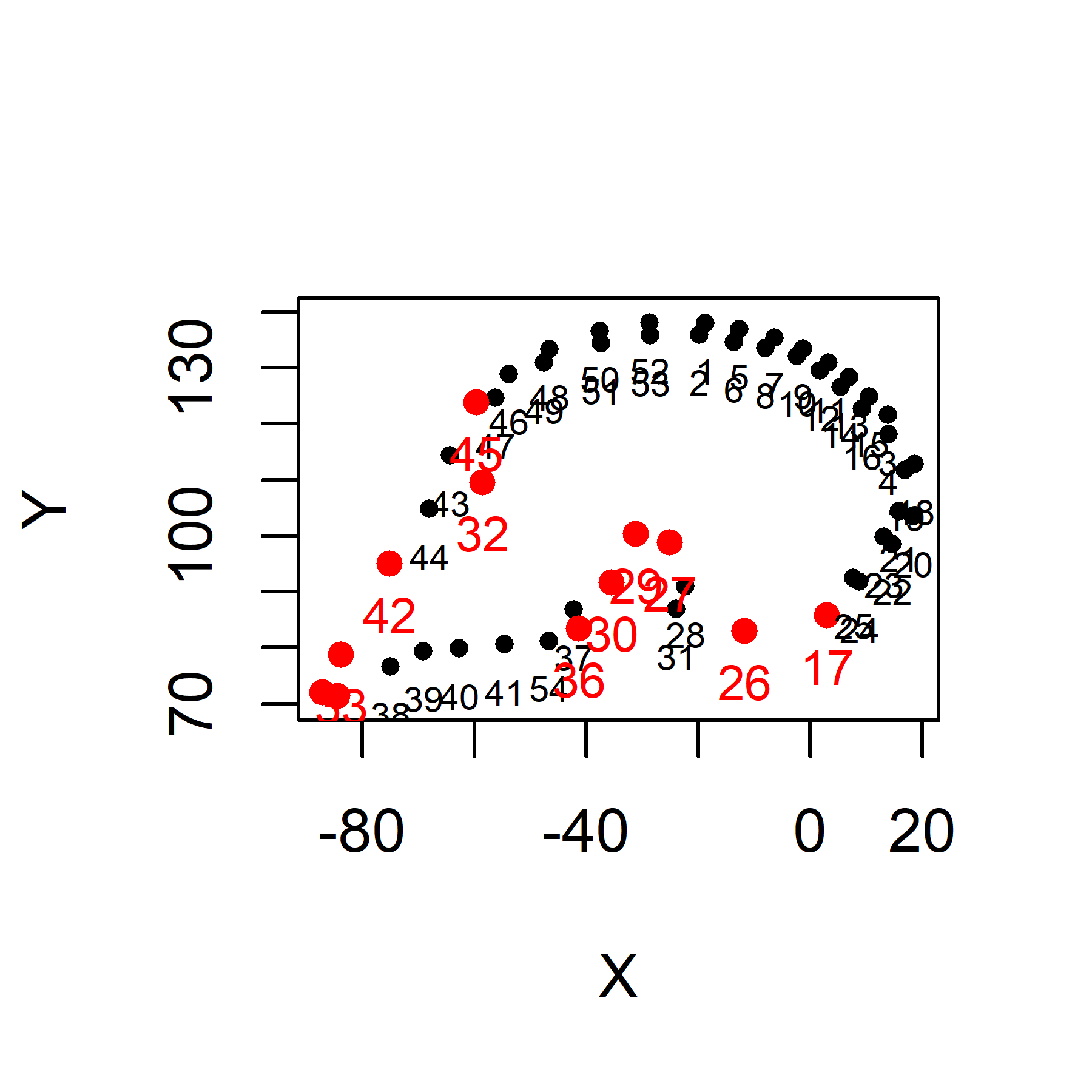Slide the 54 landmarks by minimizing bending energy. Here both slid but not Procrustes aligned outline landmarks and slid and Procrustes aligned outline landmarks are obtained.

``outline_gpa <- Morpho::procSym(outline_ar, SMvector = outline_semi_lm, outlines = outline_curves, pcAlign = FALSE)  # sliding + GPA``
``````## reflection involved
## Iteration 1 ..
## squared distance between means: 3.85834719994358
##  -------------------------------------------
## Iteration 2 ..
## squared distance between means: 0.00016856396081853
##  -------------------------------------------
## Iteration 3 ..
## squared distance between means: 1.89543101565867e-05
##  -------------------------------------------
## Iteration 4 ..
## squared distance between means: 1.67580177683664e-05
##  -------------------------------------------
## Iteration 5 ..
## squared distance between means: 1.58558336641451e-05
##  -------------------------------------------
## Iteration 6 ..
## squared distance between means: 1.49900912797382e-05
##  -------------------------------------------
## Iteration 7 ..
## squared distance between means: 1.41683205965697e-05
##  -------------------------------------------
## Iteration 8 ..
## squared distance between means: 1.34271059429379e-05
##  -------------------------------------------
## Iteration 9 ..
## squared distance between means: 1.27806248456679e-05
##  -------------------------------------------
## Iteration 10 ..
## squared distance between means: 1.22256520950769e-05
##  -------------------------------------------
## Iteration 11 ..
## squared distance between means: 1.17525231794803e-05
##  -------------------------------------------
## Iteration 12 ..
## squared distance between means: 1.13500380739102e-05
##  -------------------------------------------
## Iteration 13 ..
## squared distance between means: 1.10077043774118e-05
##  -------------------------------------------
## Iteration 14 ..
## squared distance between means: 1.07164268267304e-05
##  -------------------------------------------
## Iteration 15 ..
## squared distance between means: 1.0468634359219e-05
##  -------------------------------------------
## Iteration 16 ..
## squared distance between means: 1.02582100738881e-05
##  -------------------------------------------
## Iteration 17 ..
## squared distance between means: 1.0080379123197e-05
##  -------------------------------------------
## Iteration 18 ..
## squared distance between means: 9.93159848867143e-06
##  -------------------------------------------``````
``````outline_ar_slid <- outline_gpa\$dataslide  # slid landmarks in the original space
outline_shape <- outline_gpa\$rotated  # Procrustes coordinates
outline_shape_average <- outline_gpa\$mshape  # average shape``````

Visualize fixed (red) vs. sliding (black) landmarks for one specimen after sliding, for comparisons to the position of the semilandmarks before sliding.

``````plot(outline_ar_slid[,,1], pch = 16, col = "black", cex = 0.7, asp = 1)
points(outline_ar_slid[-outline_semi_lm,,1], pch = 16, col = "red")
text(outline_ar_slid[outline_semi_lm,,1], label = outline_semi_lm, pos = 1, col = "black", cex = 0.6)
text(outline_ar_slid[-outline_semi_lm,,1], label = c(1:k_out)[-outline_semi_lm], pos = 1, col = "red", cex = 0.8)``````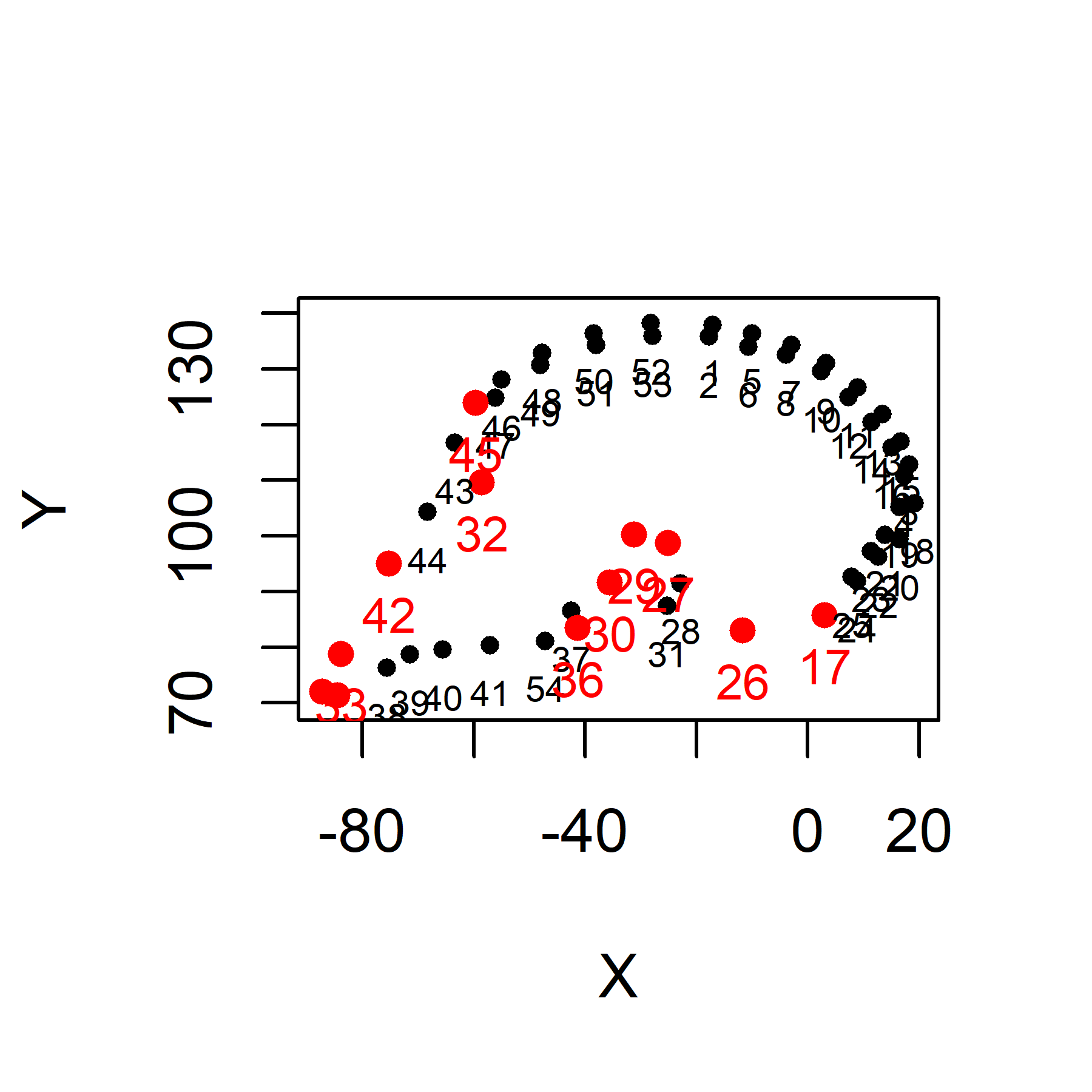For the analyses of the outline shape variation, use slid landmarks after superimposition (generalized Procrustes analysis, GPA).

Outline shape for all specimens.

``````plotAllSpecimens(outline_shape, mean = FALSE, plot.param = list(pt.cex = 0.5))
for (i in 1:n_spec) {
}``````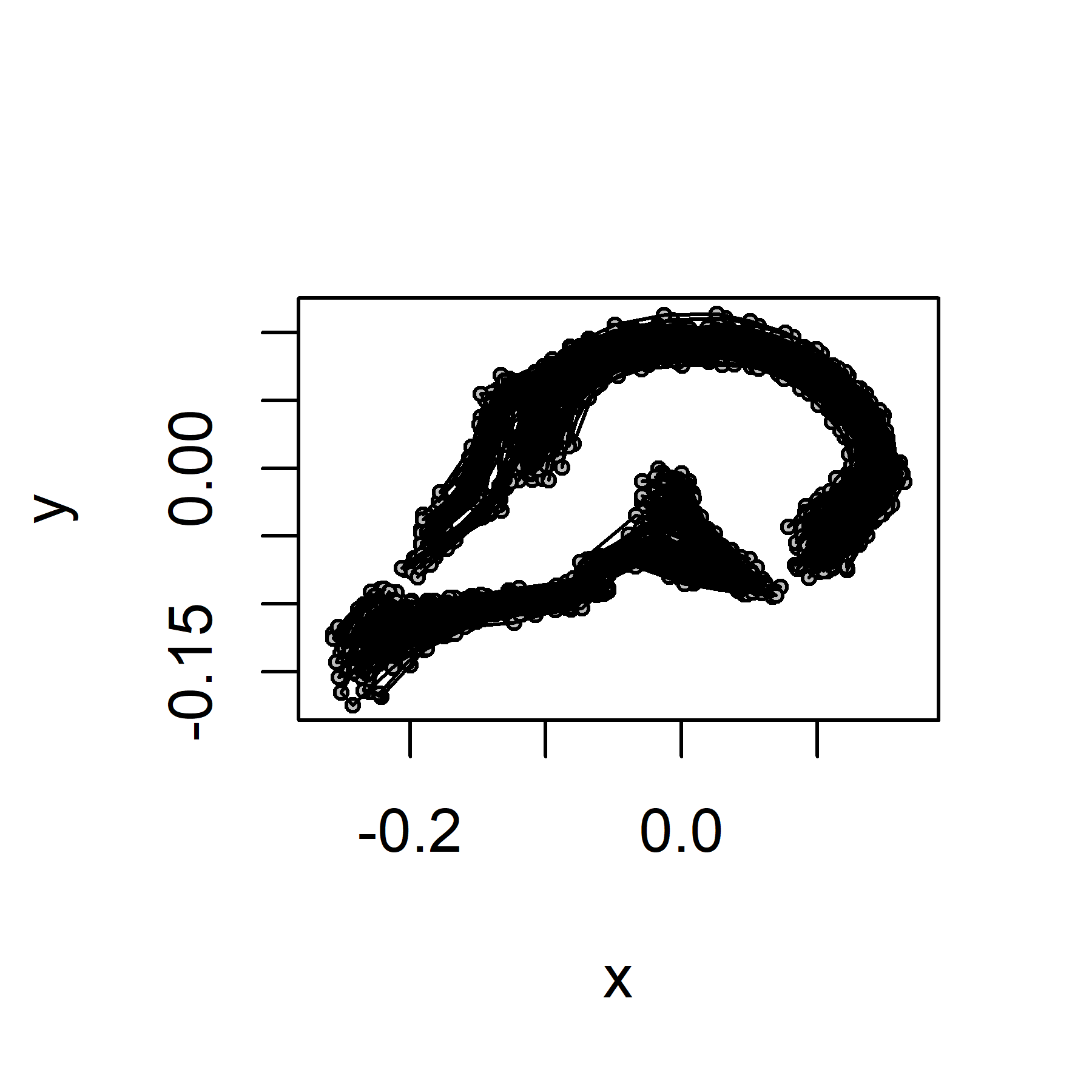Outline shape variation between species.

``````# Computation of the average outline shape for each species
outline_shape_between <- array(NA, dim = c(k_out, 2, n_species))
for (i in 1:n_species) {
spi <- which(species == levels(species)[i])
outline_shape_between[,,i] <- mshape(outline_shape[, , spi])
}
# Plot outline shape between species
plotAllSpecimens(outline_shape_between, mean = FALSE, plot.param = list(pt.cex = 0.5))
for (i in 1:n_species) {
}``````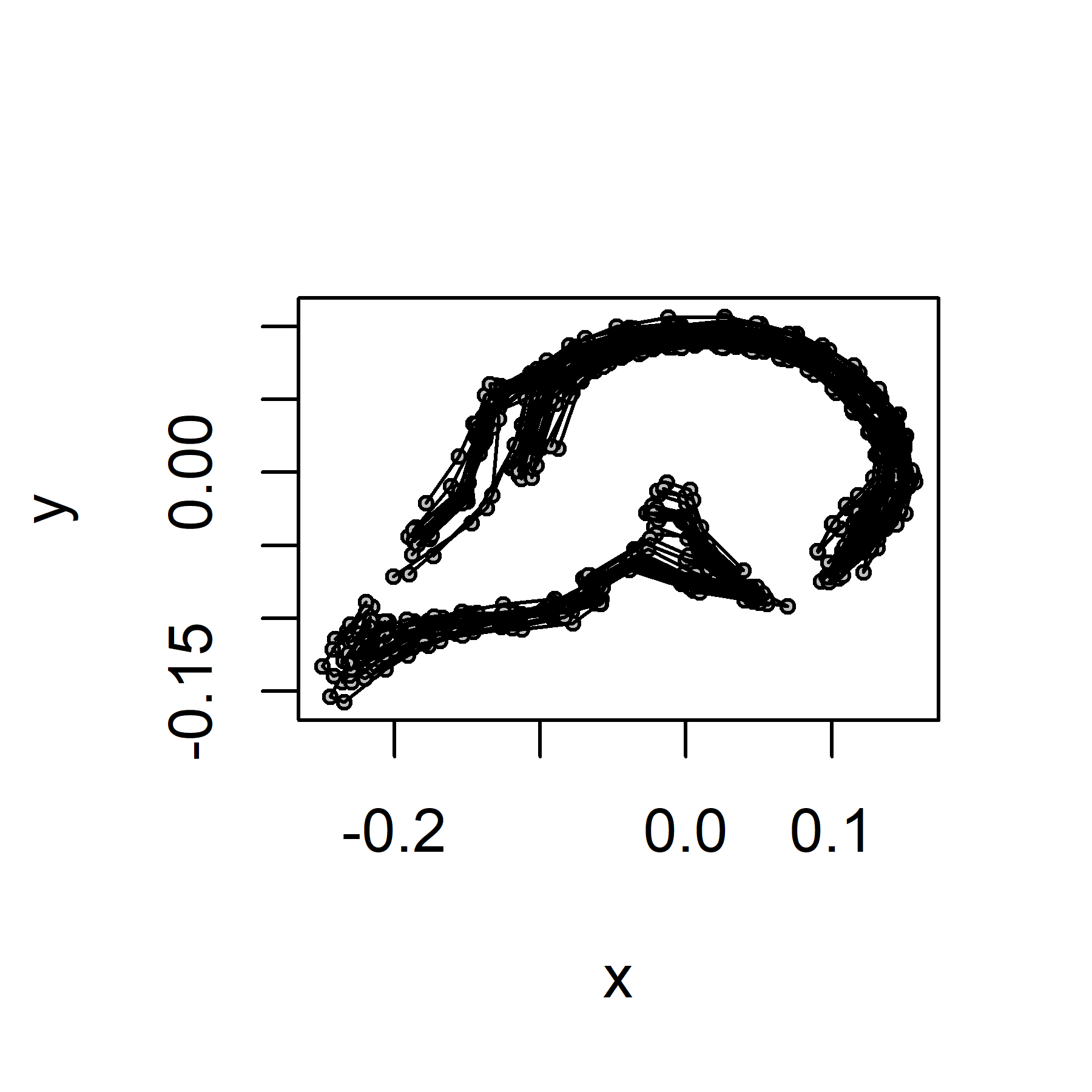Outline shape variation within species.

``````# Computation of the pooled individual within-species outline shape
outline_shape_within <- array(NA, dim = c(k_out, 2, n_spec))
for (i in 1:n_species) {
spi <- which(species == levels(species)[i])
for (j in 1:length(spi)) {
outline_shape_within[,,spi[j]] <- outline_shape[, , spi[j]] - outline_shape_between[,,i] + mshape(outline_shape_between)
}
}
# Plot the pooled individual within-species total shape
plotAllSpecimens(outline_shape_within, mean = FALSE, plot.param = list(pt.cex = 0.5))
for (i in 1:n_spec) {
}``````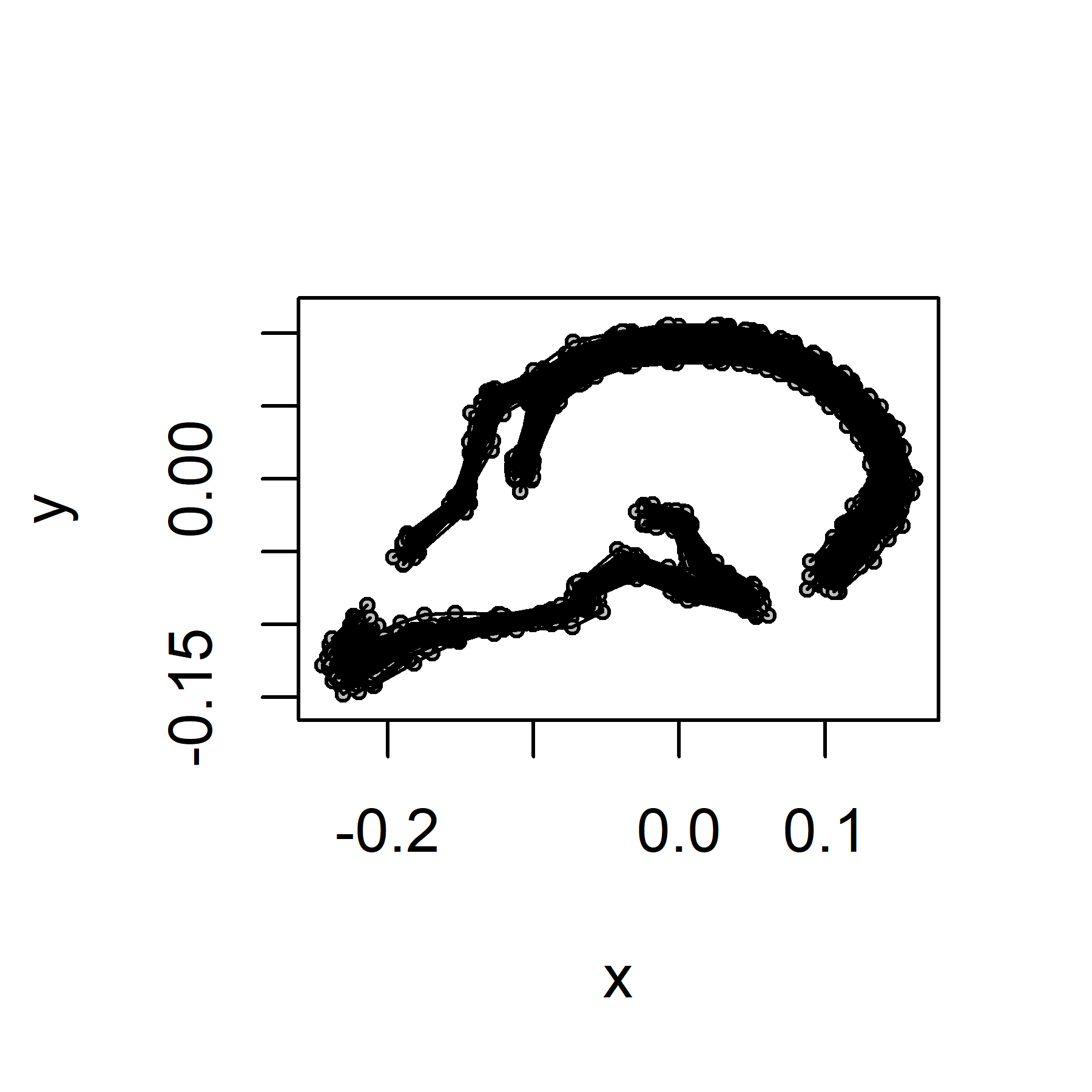For all statistical analyses of the outline shape, use slid and Procrustes aligned landmarks. Here a principal component analysis is performed.

``````# PCA
outline_shape_pca <- prcomp(two.d.array(outline_shape_between))
# Plot PC1 and PC2
col.sp <- c(rep("red", 3), rep("black", 2), rep("green", 2), rep ("blue", 8), "red", rep("green", 2))  # color vector
pch.sp <- c(rep(16, 4), 1, rep(16, 9), rep(1, 4))  # symbol vector
plot(outline_shape_pca\$x[,1:2], las = 1, pch = pch.sp, col = col.sp, asp = 1, main = "Outline shape")
text(outline_shape_pca\$x[,1:2], labels = levels(species), pos = 1, cex = 0.8)``````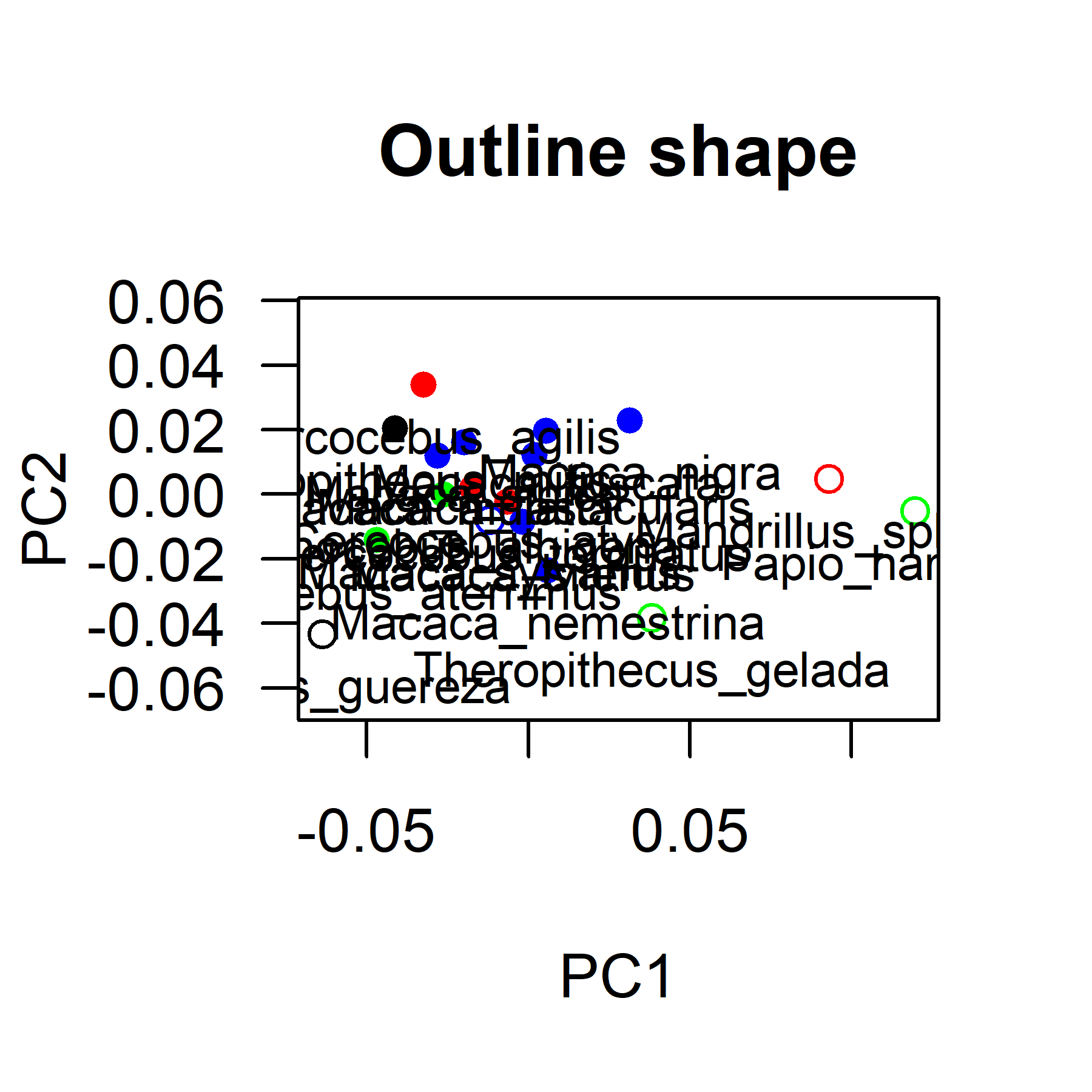## Residual shape

The 'residual' shape corresponds to the result of the warping of the 70 slid landmarks to the mean of the Procrustes aligned outline landmarks using TPS interpolation. Note that here:
- the landmark configuration to be mapped is the slid but not Procrustes aligned full landmark set (70 landmarks);
- the reference is the slid but not Procrustes aligned outline landmark subset (54 landmarks);
- the target is the mean of the slid and Procrustes aligned outline landmark subset (54 landmarks).

``residual_shape <- tps.all(papionin_ar_slid, outline_ar_slid, outline_shape_average)  # warping to the average outline shape``

For the analyses of the residual shape variation no superimposition is needed because the landmarks were already registered by the warping to the mean outline shape (in contrast to GPA, this is a non-rigid registration).

Residual shape for all specimens.

``````plotAllSpecimens(residual_shape, mean = FALSE, plot.param = list(pt.cex = 0.5))
for (i in 1:n_spec) {
}``````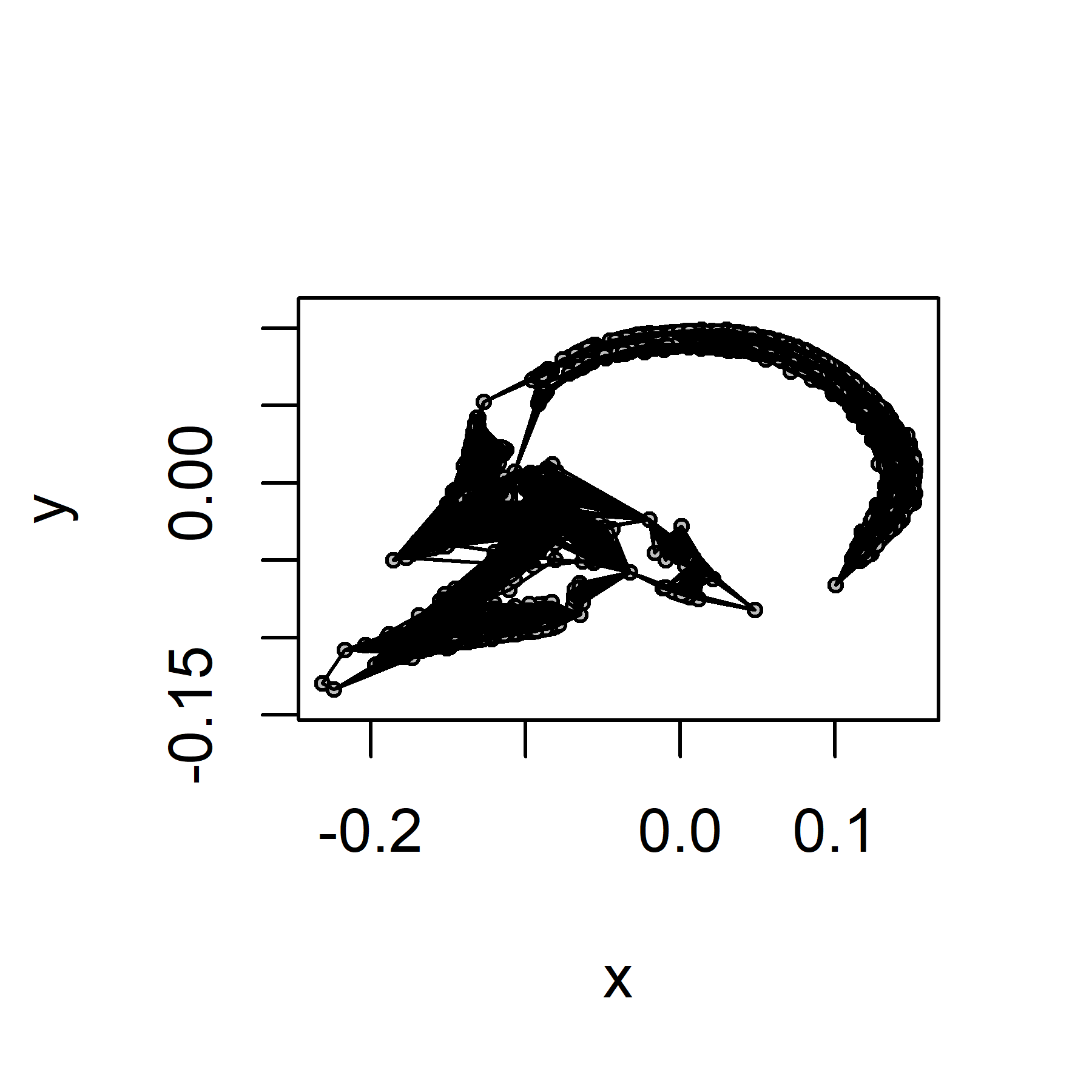Residual shape variation between species

``````# Computation of the average residual shape for each species
residual_shape_between <- array(NA, dim = c(k, 2, n_species))
for (i in 1:n_species) {
spi <- which(species == levels(species)[i])
residual_shape_between[,,i] <- mshape(residual_shape[, ,spi])
}
# Plot residual shape between species
plotAllSpecimens(residual_shape_between, mean = FALSE, plot.param = list(pt.cex = 0.5))
for (i in 1:n_species) {